The VBA Union method in Excel is designed to combine ranges into a single range. You can use VBA Union to combine multiple ranges based on a common criteria, like all positive numbers, or even use it to select a subset of a larger range.

This tutorial will introduce you to the VBA Union method and provide several examples to show you its limitations and teach you how to properly use it.

## Basic VBA Union Macro

We’re going to start this tutorial with a basic Union example. Stick with us though because soon we’ll be discussing some very important limitations of the VBA Union method.

``````Sub BasicUnionDemo()
Dim rng1 As Range
Set rng1 = Union(Range("A1:C4"), Range("E1:F4"))
rng1.Select
End Sub``````

Make powerful macros with our free VBA Developer Kit

Tutorials like this can be complicated. That’s why we created our free VBA Developer Kit and our Big Book of Excel VBA Macros to supplement this tutorial. Grab them below and you’ll be writing powerful macros in no time.

I'll take a free VBA Developer Kit

## Using VBA Union Method

When you run this macro, the ranges A1:C4 and E1:F4 are combined into one range, which we stored in variable rng1. Notice how we use the `Set` keyword to set our unified range to the variable rng1. You can’t assign the combined range to the variable without the `Set` keyword.

After the union method is applied to the ranges, the macro selects the newly combined range, so you’re left with this: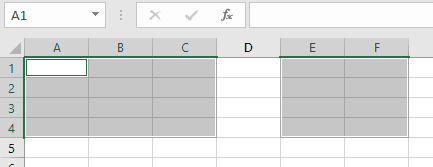It’s worthwhile to mention that the VBA Union method isn’t actually a global VBA method. It’s really a member of the Excel Type Library, so it technically should be entered like `Application.Union(...)`. Since we’re typically working directly in Excel when applying the Union method, we’re going to drop the Application and simply use the shorthand `Union(...)` notation here.

### Working with the Combined Range

Selecting the combined range is just one of many things you can do with your newly created range object. You can iterate through each item in your combined range with a simple For Loop, like this:

``````Sub BasicUnionDemo2()
Dim rng1 As Range
Dim item As Range
Set rng1 = Union(Range("A1:C4"), Range("E1:F4"))

For Each item In rng1
Next item
End Sub``````

When you run this macro, the address of each item in the range rng1 is printed to your immediate window, which you can open by pressing Ctrl+G from your VBA Editor.

```\$A\$1
\$B\$1
\$C\$1
\$A\$2
\$B\$2
\$C\$2
\$A\$3
\$B\$3
\$C\$3
\$A\$4
\$B\$4
\$C\$4
\$E\$1
\$F\$1
\$E\$2
\$F\$2
\$E\$3
\$F\$3
\$E\$4
\$F\$4
```

### Select Subset of a Range with VBA Union

One creative use for the VBA Union method is to use it to select a subset of cells in a range based on common criteria. For example, let’s say we wanted to store all the positive numbers in a column to a single variable. How would you do that?

One way to do it is to iterate through each item in the column and apply the union method to each new positive number you encounter. There are simpler ways to do this, but we’re here to demonstrate the VBA Union method.

To start, assume we have this dataset in our spreadsheet.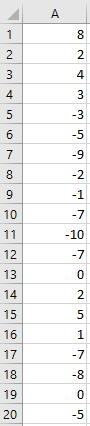We’re going to loop through each row in this column and store each positive number in a shared range. To make things interesting, we’re actually going to use Union to store all zeroes in a range, all positive numbers in a range, and all negative numbers in a range. That way, you can see the true power of organizing your data into separate ranges using the Union method. Doing it this way will also highlight some of the limitations of the Union method.

Take a look at this macro:

#### Store numbers in different ranges based on value

``````Sub VBAUnionDemo()
Dim rngPOSITIVE As Range
Dim rngNEGATIVE As Range
Dim rngZERO As Range
Dim LastRow As Long
Dim i As Long

LastRow = Range("A" & Rows.Count).End(xlUp).Row
'categorize our ranges
For i = 1 To LastRow
If IsNumeric(Range("A" & i)) Then
If Range("A" & i) > 0 Then
If rngPOSITIVE Is Nothing Then
Set rngPOSITIVE = Range("A" & i)
Else
Set rngPOSITIVE = Union(Range("A" & i), rngPOSITIVE)
End If
ElseIf Range("A" & i) < 0 Then
If rngNEGATIVE Is Nothing Then
Set rngNEGATIVE = Range("A" & i)
Else
Set rngNEGATIVE = Union(Range("A" & i), rngNEGATIVE)
End If
Else 'equals zero
If rngZERO Is Nothing Then
Set rngZERO = Range("A" & i)
Else
Set rngZERO = Union(Range("A" & i), rngZERO)
End If
End If
End If
Next i

'post-process our ranges
rngPOSITIVE.Select
rngNEGATIVE.Font.Color = vbRed
rngZERO.Font.Italic = True
End Sub``````

In this example, we use the VBA IsNumeric function to check if a cell is a number. If it is, we then categorize it based on value (greater than 0, less than 0, equal to 0).

Again, there are definitely quicker ways to produce results like this, but we’re here to demonstrate how you can use the Union method in your own macros. Once you run this macro, your final column will look like this: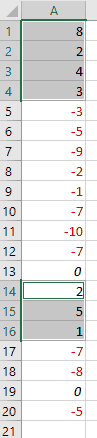Negative values will be red, cells equal to zero will be italicized, and all positive values will be selected.

## VBA Union Limitations

### Undefined (Nothing) parameters

The macro above highlights one of the primary limitations of the VBA Union method. Notice how we have an IF statement like this after testing the value of each cell:

``If rngPOSITIVE Is Nothing Then``

We have to perform this check because the Union method can’t combine ranges if one of the ranges doesn’t exist. In other words, until we define rngPOSITIVE the first time, we can’t include it in our Union statement.

If we try to include a range equal to `Nothing` in a Union expression, we’ll get an “invalid procedure call or argument” error: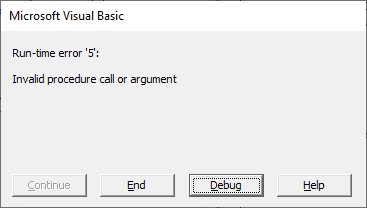The first time you encounter a cell fitting your criteria, you have to add it to your range the traditional way, like this:

``Set rngPOSITIVE = Range("A" & i)``

After the range is defined the first time, you can add to the existing range with the Union command.

### VBA Union on overlapping ranges

The second limitation deals with duplicates in a range. It’s important to point out that the VBA Union method is not the same as the mathematical Union operation. If the ranges you want to combine intersect, VBA Union will list the intersecting ranges twice. Take the following macro, for example.

``````Sub UnionDemoIntersection()
Dim rng1 As Range
Dim item As Range
Set rng1 = Union(Range("A1:B3"), Range("B2:C4"))
rng1.Select

For Each item In rng1
Next item
End Sub``````

In this example, the two ranges overlap, which is obvious when you select the combined range: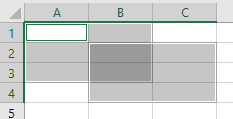Now take a look at you immediate window. You’ll notice that B2 and B3 are printed twice.

```\$A\$1
\$B\$1
\$A\$2
\$B\$2
\$A\$3
\$B\$3
\$B\$2
\$C\$2
\$B\$3
\$C\$3
\$B\$4
\$C\$4
```

Because the intersecting ranges are included in your range twice, you’ll need to be careful when using the combined range in your macro.

## Closing Thoughts

I use the VBA Union method often when I want to combine all the cells meeting a complex criteria into a common range. How do you plan on using the VBA Union method?

I hope you’ll take a minute to subscribe for more VBA tips. Simply fill out the form below and we’ll share our best time-saving VBA tips.